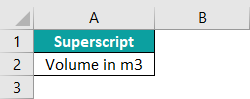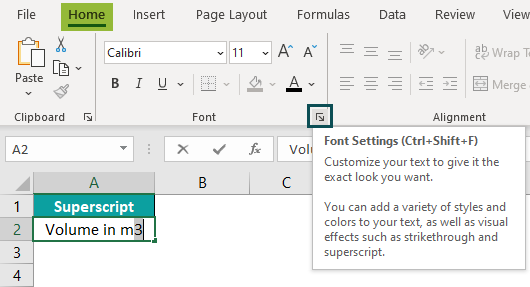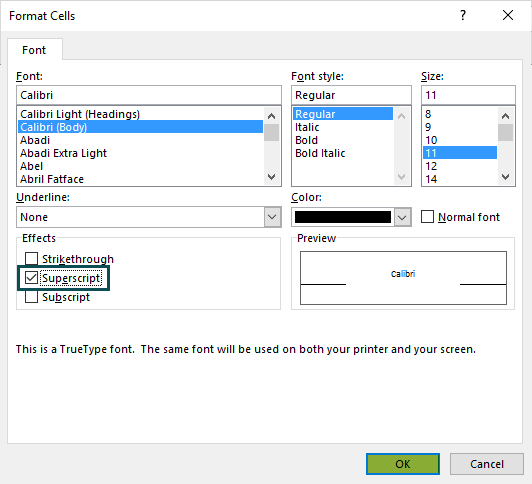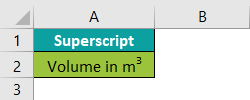# Superscript in ExcelArticle byExcelMojo Team## What Is Superscript In Excel?

The Superscript in Excel is a small letter or number typed above the baseline. If there is any preceding text in a cell, the superscript is attached to the top of the regular-sized characters. Generally, the Superscript in Excel function is used to insert Mathematical and Scientific Equations. The Superscript is available under the Home tab.

In simple words, Superscript is the text or numbers written as square units, such as a2, b2, or c2 or ordinary numbers such as 1st, 2nd, or 3rd. We use the Superscript feature to display the content in the right manner. For example, let us insert Superscript in Excel using the following steps.

• To start with, go to the Home tab and then, click on the arrow button present in the right-end corner of the Font group.
• Next, the Format Cells window opens up.
• Then, set the Font and the Font style in the Font tab.
• Similarly, set the Size of the font in the Size list.
• Next, select the Superscript checkbox in the Effects list, and click OK.

The value entered in columns A & B is the Superscript value as shown in the below image. Likewise, we can insert superscript in Excel to make our data clear and precise.

###### Key Takeaways
• The Superscript in Excel is used to put the number or text in small fonts above the baseline of numbers and text.
• The Superscript feature is used to insert values in mathematical formulas and scientific data.
• We cannot add superscript in Excel directly to individual number values as it converts the number value into string. So, we should add ‘^’ symbol to convert number into superscript.
• The Subscript is similar to the Superscript feature.

### How To Add Superscript In Excel?

We can add superscript in Excel using the below methods.

#### #1 – Long Method

For example, consider the below table with a value in cell A2. Now, let us use the following steps to apply superscript in Excel using the Long Method.

The steps to apply Superscript in Excel using long method are as follows:

Step 1: First, enter the data in the worksheet.

Step 2: Next, select the number we want as superscript; In this case, the selected number is 2.

Step 3: Then, go to the Home tab.

Step 4: Next, click the arrow button present in the right-end corner of the Font group.

Step 5: The window called Format Cells pops up.

Step 6: Next, set the Font, Font style, and Size in the Font, Font style: and Size: boxes, respectively.

Step 7: Then, select the Superscript checkbox in the Effects list.

Step 8: Click OK.

The value entered in cell A2 is the Superscript value.

Similarly, we can apply superscript in excel.

#### #2 – Shortcut Method

The following table shows a value in cell A2. Now, let us learn how to add Superscript in Excel Shortcut Method to make the data appear correctly.

We can insert Superscript in Excel shortcut method using the following steps:

Step 1: To begin with, enter the data in the worksheet.

Step 2: Next, select the number we want as Superscript; in this case, the selected value is ‘st.’

Step 3: Then, press the shortcut keys to open the Format cells window.

Step 4: Similarly, press the shortcut keys to select the Superscript option from the Effects list.

Step 5: Lastly, press Enter key; the value entered in cell A2 is the Superscript value.

Likewise, we can insert superscript in Excel.

#### #3 – Equation Method

There is another method for adding Superscripts in Excel that allows us to create Equations with proper mathematical notation, including both Subscripts and Superscripts options in Excel. This feature creates an equation inside a cell. It will create an equation that floats above the character’s baseline or number, similar to a shape or image.

The steps used to add superscript in Excel are as follows:

Step 1: First, go to the Insert tab.

Step 2: Next, click the Symbols option and select Equation.

Step 3: The Equation tab appears.

Step 4: The Type equation here box pops up on the screen.

Step 5: Then, select the Script option from the Operator group.

Step 6: The Superscript option gets selected.

Step 7: Next, enter the values in the Type equation here box.

The entered value appears in superscript format.

Thus, we can use superscript in excel.

#### #4 – Symbol Method

There is another method for adding Superscripts in Excel that allows users use symbols with proper mathematical notation, including both Subscripts and Superscripts options in Excel. This feature creates an equation inside a cell. This method uses special characters for subscripts and superscripts. Even though the function has limited character choice, all the numbers and a few symbols are available.

Step 1: To begin with, go to the Insert tab.

Step 2: Next, click the Symbols option.

Step 3: Then, select the Symbol from the options.

Step 4: The Equation tab opens.

Step 5: The window called Symbol pops up.

Step 6: Next, set Font to (normal text), and Subset to Superscripts and Subscripts.

Step 7: The selected Symbols in the box are the Superscript Symbols, as shown in the below image.

Then, click the Insert button.

Likewise, we can use Superscript in excel.

#### #5 – Type Superscript With ALT Key

To type Superscript with ALT Keys, we can use the numbers 1, 2, and 3 only. To do this, we must press some numbers while pressing the Alt key.

The below image with the keyboard excel shortcuts will only work on the Calibri or Arial fonts. We need to select this font only; if not, the characters may differ in other fonts, which we will get after typing these codes.

#### #6 – CHAR Function Method

In the below table, we have entered numbers and will apply the CHAR function to make the Superscript of the numbers.

In the table,

• Column A contains the CHAR function formulas.
• Column B contains the number to make Superscript.

The steps to do Superscript in Excel technique using CHAR function are as follows:

Step 1: First, enter the CHAR function with set numbers, as shown in column A.

Step 2: Next, we can see that the Superscript function has displayed the results in column C using the CHAR function, as shown in the below image.

### Important Things to Note

• The Superscript is a text formatting function in excel. It converts the number or text above the specified string.
• We can insert Superscript in Excel in multiple ways, such as long method, shortcut method, equation method, symbol method, etc.,
• The number format does not support some of the ways of using Superscript in Excel.
• The Superscript used in Excel is slightly different because it changes numbers to strings. If we want to make calculations, we need to use the ‘^’ operator to get the power effect.

Why is Superscript Not Working in Excel?

The Superscript puts the number or text in small fonts above the baseline of numbers, words, or characters. Generally, the Superscript feature in Excel is used in mathematical and scientific equations. Also, the equations are written exponentially.

The Superscript does not work if it is inserted in the Format Cells; Or, the Superscripts feature checkbox is left unchecked. The Superscript is also not enabled in the Format Cells window.

What are Subscript and Superscript in Excel?

The Superscript in Excel is a text or number typed above the baseline of any number, word, or character. It is presented in a smaller font than the actual number or character.

The Subscript in Excel is a text or number typed below the baseline of any number, word, or character. It is presented in a smaller font than the actual number or character.

Both of these features are mainly used in mathematical and scientific equations. Both the features can be used together in the Equation option from the Symbols option of the Insert tab.

How Superscript works in excel?

Let us learn how superscript in excel works with an example. In the following table, cell A2 has value. Now, we will apply the Superscript in excel function.The steps to do Superscript in Excel technique on text are as follows:

Step 1: First, select the number we want to make Superscript; in this case, the selected number is 3.

Step 2: Next, go to the Home tab.

Step 3: Then, click the arrow button present in the right-end corner of the Font group.Step 4: The window called Format Cells pops up.

Step 5: Now, set the Font in the Font list, set the Font style in the Font style list, and also set the Size of the font in the Size list.

Step 6: Next, select the Superscript checkbox in the Effects list.

Step 7: Then, click OK.Step 8: Finally, press the Enter key; the value entered in cell A2 is displayed as the Superscript value as ‘Volume in m3’, as shown in the below image.Likewise, we can use Superscript in excel to insert superscript values.

Where is Superscript in Excel?

We can insert Superscript in Excel using the below steps:

1. First, go to the Home tab.
2. Next, click the arrow button present in the right-end corner of the Font group.
3. The window called Format Cells pops up.
4. Then, set the Font, the Font style and the Size in the Font tab.
5. Now, select the Superscript checkbox in the Effects list.
6. Click OK.